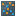You are viewing the RapidMiner Studio documentation for version 8.0 - Check here for latest version

#Cluster Count Performance (RapidMiner Studio Core)

## Synopsis

This operator creates a performance vector containing the 'Number of clusters' and 'Cluster Number Index' criteria from a cluster model.

## Description

This is a very simple operator. It takes a cluster model as input and returns a performance vector that has the 'Number of clusters' and 'Cluster Number Index' criteria. The 'Number of clusters' criteria contains the number of clusters. The 'Cluster Number Index' criteria builds a derived index from the number of clusters by using the formula 1 - (k / n) with k as thw number of clusters and n as the number of examples. This can be used for optimizing the coverage of a cluster result with respect to the number of clusters. Optionally, a performance vector can be provided as input as well. In that case the 'Number of clusters' and 'Cluster Number Index' criteria are appended to the given performance vector.

## Input

•cluster model (Cluster Model)

This input port expects a cluster model. It is the output of the Subprocess operator in the attached Example Process.

•performance (Performance Vector)

This optional port expects a performance vector. A performance vector is a list of performance criteria values.

## Output

•cluster model (Cluster Model)

The cluster model that was given as input is passed without any modifications to the output through this port. This is usually used to reuse the same cluster model in further operators or to view the cluster model in the Results Workspace.

•performance (Performance Vector)

The performance vector containing the 'Number of clusters' and 'Cluster Number Index' criteria is returned through this port.

## Tutorial Processes

### Generating a performance vector with the 'Number of clusters' criteria

This Example Process starts with the Subprocess operator. The subprocess delivers a cluster model and a performance vector. A breakpoint is inserted here so that you can have a look at the cluster model. You can see that the cluster model has two clusters. This cluster model is provided as input to the Cluster Count Performance operator which returns a performance vector with the 'Number of clusters' criteria. As there were two clusters in the given cluster model, the 'Number of clusters' criteria has value 2. Now connect the second output port of the Subprocess operator to the performance input port of the Cluster Count Performance operator. Run the process again, you will see that this time the 'Number of clusters' parameter is appended to the given performance vector.